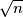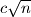### IMO 2014 problem 6

Kvaliteta:
Avg: 0,0
Težina:
Avg: 0,0
A set of line sin the plane is in general position if no two are parallel and no three pass through the same point. A set of lines in general position cuts the plane into regions, some of which have finite area; we call these its finite regions. Prove that for all sufficiently large$n$, in any set of$n$ lines in general position it is possible to colour at least$\sqrt{n}$ of the lines blue in such a way that none of its finite regions has a completely blue boundary.

Note: Results with$\sqrt{n}$ replaced by$c \sqrt{n}$ will be awarded points depending on the value of the constant$c$.
Izvor: International Mathematical Olympiad 2014, day 2